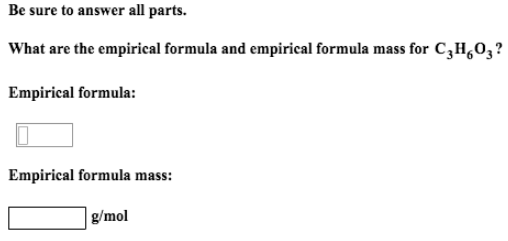# Problem: Be sure to answer all parts.What are the empirical formula and empirical formula mass for C 3H6O3?

###### FREE Expert Solution
82% (178 ratings)###### Problem Details

Be sure to answer all parts.

What are the empirical formula and empirical formula mass for C 3H6O3Frequently Asked Questions

What scientific concept do you need to know in order to solve this problem?

Our tutors have indicated that to solve this problem you will need to apply the Empirical Formula concept. You can view video lessons to learn Empirical Formula. Or if you need more Empirical Formula practice, you can also practice Empirical Formula practice problems.